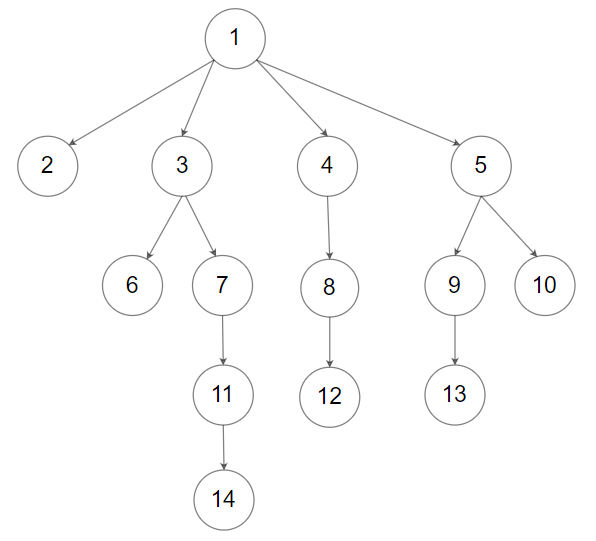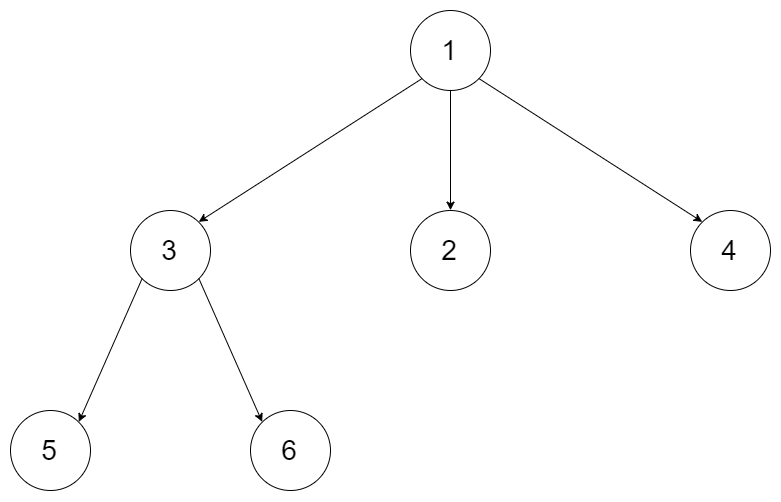##### Welcome to Subscribe On Youtube

Formatted question description: https://leetcode.ca/all/1506.html

# 1506. Find Root of N-Ary Tree

Medium

## Description

Given all the nodes of an N-ary tree as an array Node[] tree where each node has a unique value.

Find and return the root of the N-ary tree.

Could you solve this problem in constant space complexity with a linear time algorithm?

Nary-Tree input serialization is represented in their level order traversal, each group of children is separated by the null value (See examples).For example, the above tree is serialized as [1,null,2,3,4,5,null,null,6,7,null,8,null,9,10,null,null,11,null,12,null,13,null,null,14].

Custom testing:

You should provide the serialization of the input tree.

The Driver code then extracts the nodes from the tree and shuffles them. You shouldn’t care how the extracted nodes are shuffled.

The driver code will provide you with an array of the extracted nodes in random order and you need to find the root of the tree out of these nodes.

Example 1:Input: tree = [1,null,3,2,4,null,5,6]

Output: [1,null,3,2,4,null,5,6]

Explanation: The input tree is shown above. The driver code first extracts the nodes so we now have an array of all tree nodes [Node(1),Node(3),Node(2),Node(4),Node(5),Node(6)], then the array is randomly shuffled, thus the actual input is [Node(5),Node(4),Node(3),Node(6),Node(2),Node(1)].

The root of the tree is Node(1) and the output is the serialization of the node you return.

Example 2:Input: tree = [1,null,2,3,4,5,null,null,6,7,null,8,null,9,10,null,null,11,null,12,null,13,null,null,14] Output: [1,null,2,3,4,5,null,null,6,7,null,8,null,9,10,null,null,11,null,12,null,13,null,null,14]

Constraints:

• The total number of nodes is between [1, 5 * 10^4].
• Each node has a unique value.

## Solution

A straightforward solution is to use a map to store each node’s parent when looping over all nodes for the first time. Then loop over all nodes again and find the node that does not have a parent, which is the root. This solution uses O(n) time complexity and O(n) space complexity.

To solve this problem in O(n) time complexity and O(1) space complexity, use the fact that each node has a unique value. Therefore, use bitwise XOR to solve this problem.

Use xor to store the result of bitwise XOR of all nodes’ values. Loop over all nodes and calculate the bitwise XOR result of all nodes. For each node that is also a child, calculate its value again to the bitwise XOR result. Finally, only the root is calculated once in the bitwise XOR result, and all the other nodes are calculated twice in the bitwise XOR result, so the bitwise XOR result equals the root’s value. Then loop over all nodes again and find the node that has the value equal to the root’s value, which is the root, and return the root.

• /*
// Definition for a Node.
class Node {
public int val;
public List<Node> children;

public Node() {
children = new ArrayList<Node>();
}

public Node(int _val) {
val = _val;
children = new ArrayList<Node>();
}

public Node(int _val,ArrayList<Node> _children) {
val = _val;
children = _children;
}
};
*/

class Solution {
public Node findRoot(List<Node> tree) {
int xor = 0;
for (Node node : tree) {
xor ^= node.val;
List<Node> children = node.children;
for (Node child : children)
xor ^= child.val;
}
for (Node node : tree) {
if (node.val == xor)
return node;
}
return null;
}
}

############

/*
// Definition for a Node.
class Node {
public int val;
public List<Node> children;

public Node() {
children = new ArrayList<Node>();
}

public Node(int _val) {
val = _val;
children = new ArrayList<Node>();
}

public Node(int _val,ArrayList<Node> _children) {
val = _val;
children = _children;
}
};
*/

class Solution {
public Node findRoot(List<Node> tree) {
int x = 0;
for (Node node : tree) {
x ^= node.val;
for (Node child : node.children) {
x ^= child.val;
}
}
for (int i = 0;; ++i) {
if (tree.get(i).val == x) {
return tree.get(i);
}
}
}
}

• // OJ: https://leetcode.com/problems/find-root-of-n-ary-tree/
// Time: O(N)
// Space: O(N)
class Solution {
public:
Node* findRoot(vector<Node*> A) {
unordered_set<Node*> s(begin(A), end(A));
for (auto &n : A) {
for (auto &c : n->children) s.erase(c);
}
return *s.begin();
}
};

• """
# Definition for a Node.
class Node:
def __init__(self, val=None, children=None):
self.val = val
self.children = children if children is not None else []
"""

class Solution:
def findRoot(self, tree: List['Node']) -> 'Node':
xorsum = 0
for node in tree:
xorsum ^= node.val
for child in node.children:
xorsum ^= child.val

for node in tree:
if node.val == xorsum:
return node


• /**
* Definition for a Node.
* type Node struct {
*     Val int
*     Children []*Node
* }
*/

func findRoot(tree []*Node) *Node {
x := 0
for _, node := range tree {
x ^= node.Val
for _, child := range node.Children {
x ^= child.Val
}
}
for i := 0; ; i++ {
if tree[i].Val == x {
return tree[i]
}
}
}

• /**
* Definition for Node.
* class Node {
*     val: number
*     children: Node[]
*     constructor(val?: number, children?: Node[]) {
*         this.val = (val===undefined ? 0 : val)
*         this.children = (children===undefined ? [] : children)
*     }
* }
*/

function findRoot(tree: Node[]): Node | null {
let x = 0;
for (const node of tree) {
x ^= node.val;
for (const child of node.children) {
x ^= child.val;
}
}
return tree.find(node => node.val === x) || null;
}